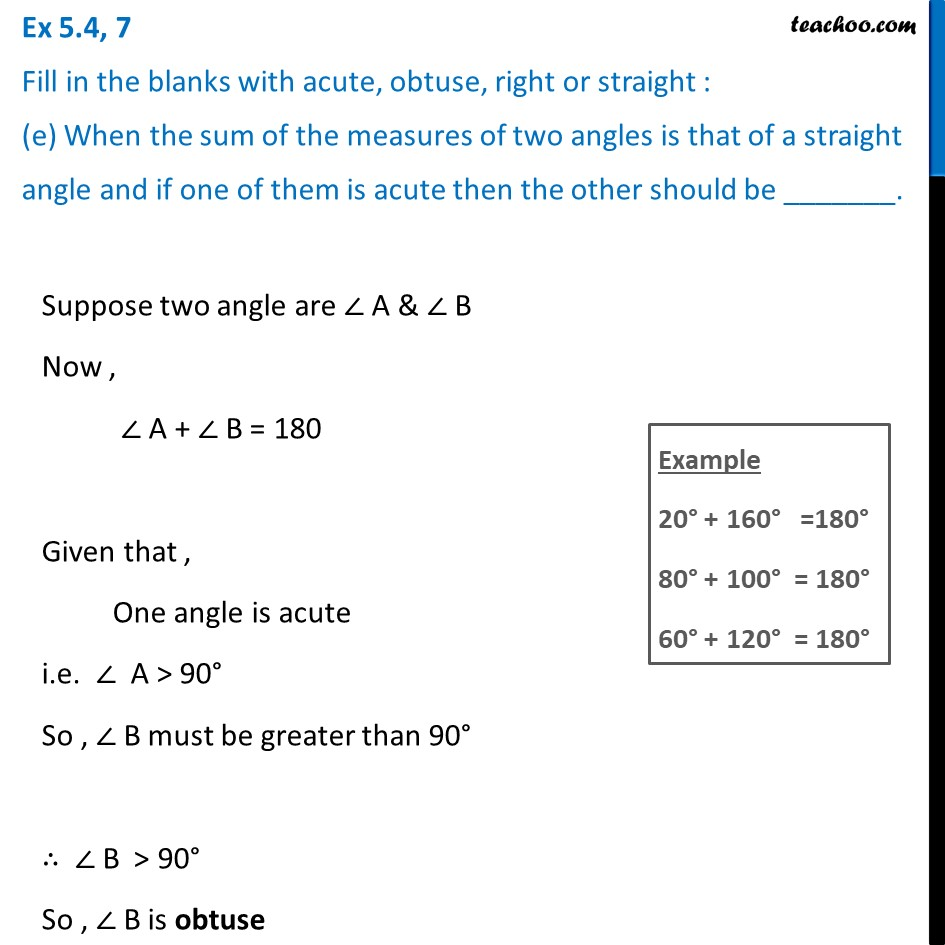1. Chapter 5 Class 6 Understanding Elementary Shapes
2. Serial order wise
3. Ex 5.4

Transcript

Ex 5.4, 7 Fill in the blanks with acute, obtuse, right or straight : (e) When the sum of the measures of two angles is that of a straight angle and if one of them is acute then the other should be _______. Suppose two angle are ∠ A & ∠ B Now , ∠ A + ∠ B = 180 Given that , One angle is acute i.e. ∠ A > 90° So , ∠ B must be greater than 90° ∴ ∠ B > 90° So , ∠ B is obtuse